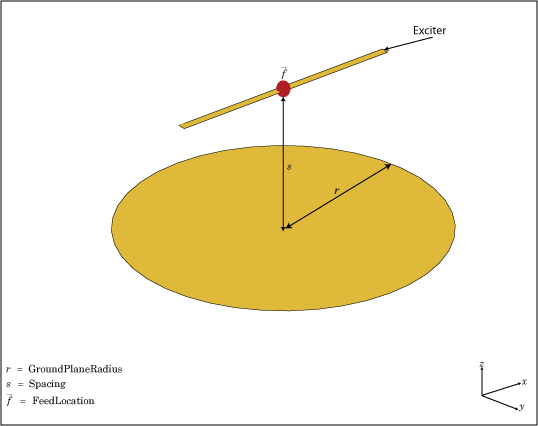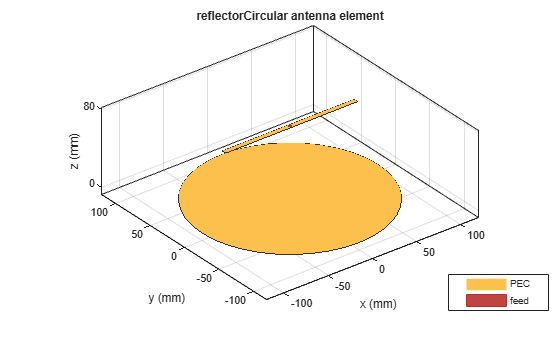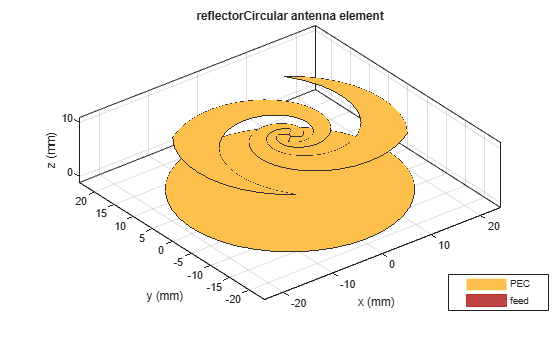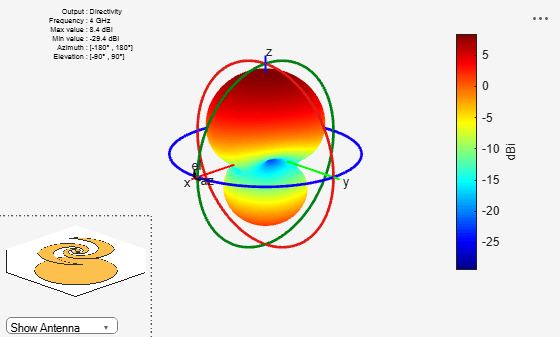# reflectorCircular

Create circular reflector-backed antenna

## Description

Use the `reflectorCircular` object to create a circular reflector-backed antenna. By default the exciter is a dipole. The dimensions are chosen for an operating frequency of 1 GHz.## Creation

### Syntax

``rc = reflectorCircular``
``rc = reflectorCircular(Name,Value)``

### Description

example

````rc = reflectorCircular` creates a circular reflector backed antenna. ```

example

````rc = reflectorCircular(Name,Value)` sets properties using one or more name-value pair. For example, ```rc = reflectorCircular('Radius',0.2)``` creates a circular reflector of radius 0.2 m. Enclose each property name in quotes.```

## Properties

expand all

Antenna type used as an exciter, specified as an object. Except for reflector and cavity antenna elements, you can use all the single elements in the Antenna Toolbox™ as an exciter.

Example: `'Exciter',spiralEquiangular`

Example: `rc.Exciter = spiralEquiangular`

Radius of reflector, specified as a scalar in meters.

Example: `'Radius',0.2`

Example: `rc.Radius = 0.2`

Data Types: `double`

Distance between the exciter and the reflector, specified as a scalar in meters.

Example: `'Spacing',7.5e-2`

Example: `rc.Spacing = 7.5e-2`

Data Types: `double`

Type of dielectric material used as a substrate, specified as an object. For more information see, `dielectric`. For more information on dielectric substrate meshing, see Meshing.

Note

The substrate dimensions must be equal to the groundplane dimensions.

Example: ```d = dielectric('FR4'); 'Substrate',d```

Example: ```d = dielectric('FR4'); rc.Substrate = d```

Create probe feed from backing structure to exciter, specified as `0` or `1` or a scalar. By default, probe feed is not enabled.

Example: `'EnableProbeFeed',1`

Example: `rc.EnableProbeFeed = 1`

Data Types: `double` | `logical`

Lumped elements added to the antenna feed, specified as a lumped element object handle. You can add a load anywhere on the surface of the antenna. By default, the load is at the origin. For more information, see `lumpedElement`.

Example: `'Load',lumpedelement`. `lumpedelement` is the object handle for the load created using `lumpedElement`.

Example: ```rc.Load = lumpedElement('Impedance',75)```

Tilt angle of the antenna, specified as a scalar or vector with each element unit in degrees. For more information, see Rotate Antennas and Arrays.

Example: `'Tilt',90`

Example: `ant.Tilt = 90`

Example: `'Tilt',[90 90]`,`'TiltAxis',[0 1 0;0 1 1]` tilts the antenna at 90 degrees about the two axes defined by the vectors.

Note

The `wireStack` antenna object only accepts the dot method to change its properties.

Data Types: `double`

Tilt axis of the antenna, specified as:

• Three-element vector of Cartesian coordinates in meters. In this case, each coordinate in the vector starts at the origin and lies along the specified points on the X-, Y-, and Z-axes.

• Two points in space, each specified as three-element vectors of Cartesian coordinates. In this case, the antenna rotates around the line joining the two points in space.

• A string input describing simple rotations around one of the principal axes, 'X', 'Y', or 'Z'.

Example: `'TiltAxis',[0 1 0]`

Example: `'TiltAxis',[0 0 0;0 1 0]`

Example: `ant.TiltAxis = 'Z'`

Note

The `wireStack` antenna object only accepts the dot method to change its properties.

Data Types: `double`

## Object Functions

 `show` Display antenna or array structure; display shape as filled patch `axialRatio` Axial ratio of antenna `beamwidth` Beamwidth of antenna `charge` Charge distribution on metal or dielectric antenna or array surface `current` Current distribution on metal or dielectric antenna or array surface `design` Design prototype antenna or arrays for resonance at specified frequency `EHfields` Electric and magnetic fields of antennas; Embedded electric and magnetic fields of antenna element in arrays `impedance` Input impedance of antenna; scan impedance of array `mesh` Mesh properties of metal or dielectric antenna or array structure `meshconfig` Change mesh mode of antenna structure `optimize` Optimize antenna or array using SADEA optimizer `pattern` Radiation pattern and phase of antenna or array; Embedded pattern of antenna element in array `patternAzimuth` Azimuth pattern of antenna or array `patternElevation` Elevation pattern of antenna or array `returnLoss` Return loss of antenna; scan return loss of array `sparameters` S-parameter object `vswr` Voltage standing wave ratio of antenna

## Examples

collapse all

Create and view a default circular reflector backed antenna.

`rc = reflectorCircular`
```rc = reflectorCircular with properties: Exciter: [1x1 dipole] Substrate: [1x1 dielectric] GroundPlaneRadius: 0.1000 Spacing: 0.0750 EnableProbeFeed: 0 Tilt: 0 TiltAxis: [1 0 0] Load: [1x1 lumpedElement] ```
`show(rc)`Create an equiangular spiral backed by a circular reflector.

```ant = reflectorCircular('Exciter',spiralEquiangular,'GroundPlaneRadius', ... 0.02,'Spacing', 0.01); show(ant)```Plot the radiation pattern of the antenna at 4 GHz.

`pattern(ant,4e9)`